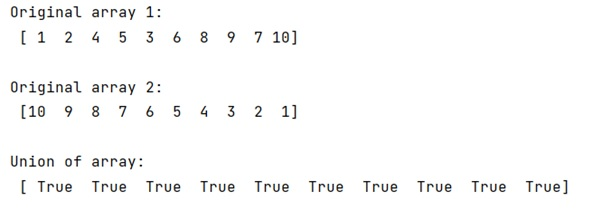# numpy.logical_or() for more than two arguments

Learn, how to save a list as NumPy array in Python?
Submitted by Pranit Sharma, on January 03, 2023

NumPy is an abbreviated form of Numerical Python. It is used for different types of scientific operations in python. Numpy is a vast library in python which is used for almost every kind of scientific or mathematical operation. It is itself an array which is a collection of various methods and functions for processing the arrays.

## Numpy `logical_or` for more than two arguments

OR operation is used as a logical operator, logically AND operator between two values is the SUM of two values and it returns TRUE only if any one of its operands or both are TRUE otherwise FALSE.

Suppose we are given two NumPy arrays and we need to find the union of two arrays. For this purpose, we will use the numpy.logical_or() method.

This will also work if we have one multi-dimensional array instead of separate arrays. But a tuple of three equal-length 1D arrays is an array-like in NumPy terms and can be used as a 2D array.
The | operator can be used as a shorthand for np.logical_or on boolean ndarrays.

Let us understand with the help of an example,

## Python code to demonstrate the example of numpy.logical_or() for more than two arguments

```# Import numpy
import numpy as np

# Creating two numpy arrays
arr1 = np.array([1,2,4,5,3,6,8,9,7,10])
arr2 = np.array([10,9,8,7,6,5,4,3,2,1])

# Display original arrays
print("Original array 1:\n",arr1,"\n")
print("Original array 2:\n",arr2,"\n")

# Find the union of these arrays
res = np.logical_or(arr1,arr2)

# Display result
print("Union of array:\n",res)
```

Output: